# Test: Control System- 3

## 25 Questions MCQ Test Basic Electronics Engineering for SSC JE (Technical) | Test: Control System- 3

Description
Attempt Test: Control System- 3 | 25 questions in 50 minutes | Mock test for Electrical Engineering (EE) preparation | Free important questions MCQ to study Basic Electronics Engineering for SSC JE (Technical) for Electrical Engineering (EE) Exam | Download free PDF with solutions
QUESTION: 1

Solution:
QUESTION: 2

Solution:
QUESTION: 3

### If the gain of the open loop system is doubled, thegain margin of the system is

Solution:
QUESTION: 4

Which one of the following is not a property ofroot loci ?

Solution:
QUESTION: 5

Match List-I (System G(s) with List-II (Nature of response) and select the correct answer using
the code given below the lists: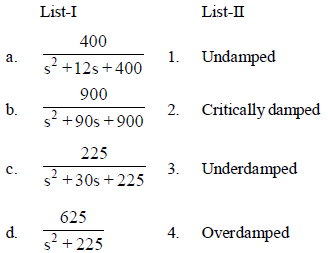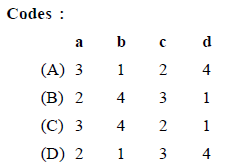Solution:
QUESTION: 6

Given a unity feedback system with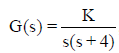what is the value of K for adamping ratio of 0.5 ?

damping ratio of 0.5 ?

Solution:
QUESTION: 7

What is the steady state error for a unity feedback control system having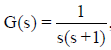due to unit ramp input ?

Solution:
QUESTION: 8

For which one of the following, given physical realization corresponds to PD controller ?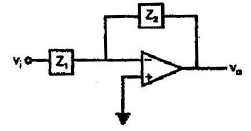Solution:
QUESTION: 9

A phase lead compensating network consists of only capacitors and resistors, The locations of its pole and zero in s -plane are at Pc and Zc respectively. Which of the following conditions must be satisf4ied ?

Solution:
QUESTION: 10

A composite R-C network yielded the following transfer function when calculated from its components :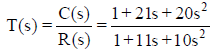The network can be used ad which one of the following ?

Solution:
QUESTION: 11

Which one of the following is a disadvantage ofproportional controller ?

Solution:
QUESTION: 12

The open loop transfer function of a unity negative feedback control system is given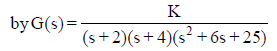Which is the value of K which causes sustainedoscillations in the closed loop system ?

Solution:
QUESTION: 13

For a stable system, what are the restrictions onthe gain margin and phase margin ?

Solution:
QUESTION: 14

For a given unity feedback system with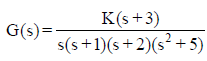axis intercept for locus asymptotes ?

Solution:
QUESTION: 15

A controller transfer function is given by C(s) =(2s+1)/ (0.2s+1). What is its nature andparameter ?

Solution:
QUESTION: 16

What is the number of root-locus segments whichdo not terminate on zeros ?

Solution:
QUESTION: 17

The characteristic polynomial of a system isq(s) = 2s5 + s4 + 4s3 + 2s5 +2s+ 1

Which one of the following is correct ?

Solution:
QUESTION: 18

How can the steady-state error in a system bereduced ?

Solution:
QUESTION: 19

For the unity feedback system with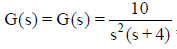,what is the steady State error resulting from an input 10t ?

Solution:
QUESTION: 20

A system with gain margin close to unity or aphase margin close to zero is

Solution:
QUESTION: 21

The open loop transfer function of a unity feedback control system is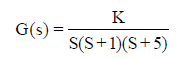What is the value of K for its stable operation ?

Solution:
QUESTION: 22

Match List-I (Shape of nyquist Plot) with List-II (grain margin) and select the correct answer using the codes given below the lists: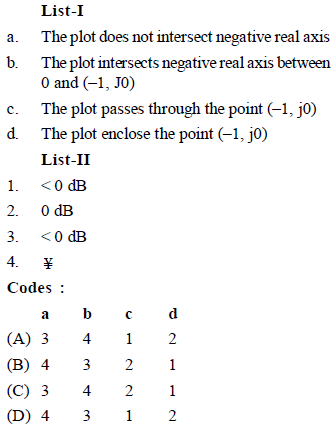Solution:
QUESTION: 23

Which one of the following is correct ?The root locus is the path of the roots of thecharacteristic equation traced out in the s-plane

Solution:
QUESTION: 24

Consider the function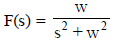is the Laplace transform of (t). What is the steady state value of f(t) ?

Solution:
QUESTION: 25

How can the bandwidth of a control system beincreased ?

Solution:Use Code STAYHOME200 and get INR 200 additional OFF Use Coupon Code